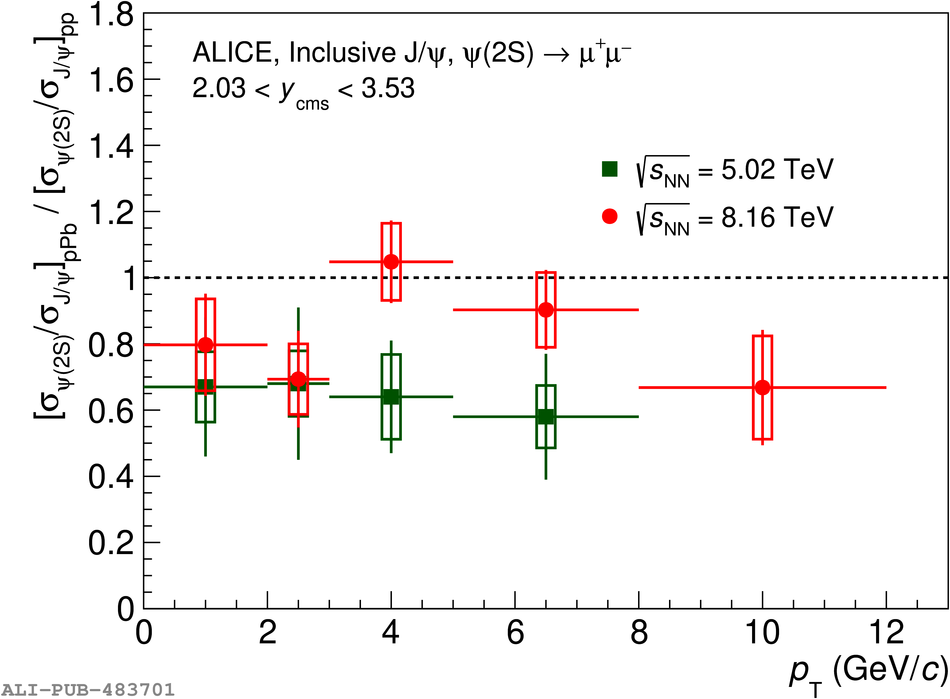# Figure 11

 Double ratio of $\psip$ and J/$\psi$ cross sections in \ppb and pp collisions as a function of transverse momentum, at forward (left) and backward (right) rapidity at $\sqrtSnn = 8.16$ TeV, compared with the corresponding results at $\sqrtSnn = 5.02$ TeV . The error bars represent the statistical uncertainties, while the boxes correspond to uncorrelated systematic uncertainties.